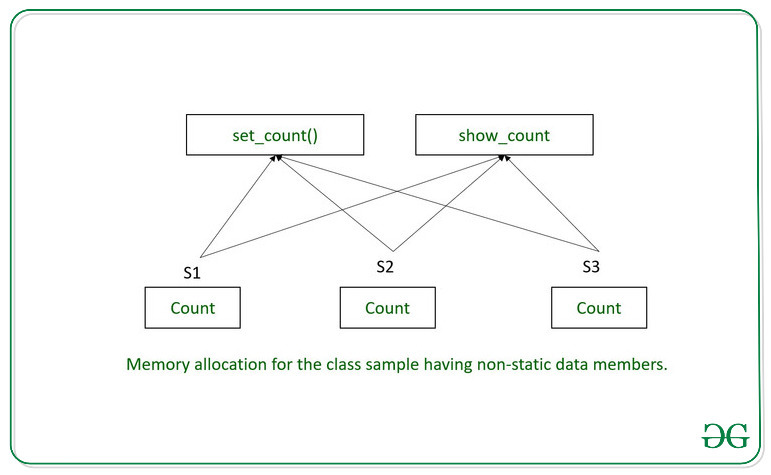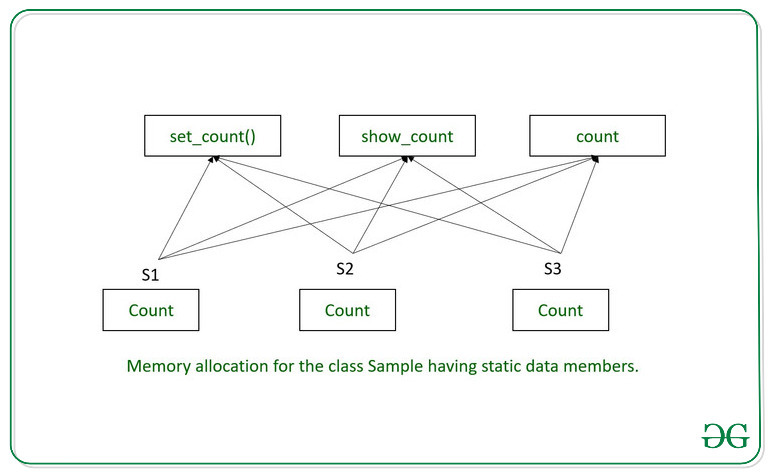# Memory Allocation in Static Data Members in C++

• Difficulty Level : Easy
• Last Updated : 14 Jun, 2020

C++ allows defining static data members within a class using the static keyword.
When a data member is declared as static, then we must keep the following note in mind:

• Irrespective of the number of objects created, only a single copy of the static member is created in memory.
• All objects of a class share the static member.
• All static data members are initiated to zero when the first object of that class is created.
• Static data members are visible only within the class but their lifetime is the entire program.

Relevance:
Static data members are usually used to maintain values that are common for the entire class. , For Example, to keep a track of how many objects of a particular class have been created.

Place of Storage:
Although static data members are declared inside a class, they are not considered to be a part of the objects. Consequently, their declaration in the class is not considered as their definition. A static data member is defined outside the class. This means that even though the static data member is declared in class scope, their definition persists in the entire file. A static member has file scope. However, since a static data member is declared inside the class, they can be accessed only by using the class name and the scope resolution operator.

Below is the program to illustrate memory allocation in static and non-static data members:

Program 1: to illustrate non-static members

 `// C++ program to illustrate``// non-static data members``using` `namespace` `std;``#include `` ` `// Class``class` `GfG {``private``:``    ``// Created a variable``    ``int` `count = 0;`` ` `public``:``    ``// Member function to increment``    ``// value of count``    ``void` `set_count()``    ``{``        ``count++;``    ``}`` ` `    ``// Member function to access the``    ``// private members of this class``    ``void` `show_count()``    ``{`` ` `        ``// print the count variable``        ``cout << count << ``'\n'``;``    ``}``};`` ` `// Driver Code``int` `main()``{``    ``// Objects of class GfG``    ``GfG S1, S2, S3;`` ` `    ``// Set count variable to 1``    ``// for each object``    ``S1.set_count();``    ``S2.set_count();``    ``S3.set_count();`` ` `    ``// Function to display count``    ``// for each object``    ``S1.show_count();``    ``S2.show_count();``    ``S3.show_count();`` ` `    ``return` `0;``}`

Output:

```1
1
1
```

Below is the illustration of memory allocation for the above program:Explanation:
All three objects of class GfG S1, S2, and S3 share the member functions but have a separate copy of the data member count. In main(), the set_count() is explicitly called to set the value of count to 1. Now, each object has the value of its count = 1.

Program 2: to illustrate static members:

 `// C++ program to illustrate``// non-static data members``using` `namespace` `std;``#include `` ` `// Class``class` `GfG {``private``:``    ``// Created a static variable``    ``static` `int` `count;`` ` `public``:``    ``// Member function to increment``    ``// value of count``    ``void` `set_count()``    ``{``        ``count++;``    ``}`` ` `    ``// Member function to access the``    ``// private members of this class``    ``void` `show_count()``    ``{`` ` `        ``// print the count variable``        ``cout << count << ``'\n'``;``    ``}``};`` ` `int` `GfG::count = 0;`` ` `// Driver Code``int` `main()``{``    ``// Objects of class GfG``    ``GfG S1, S2, S3;`` ` `    ``// Increment count variable``    ``// by 1 for each object``    ``S1.set_count();``    ``S2.set_count();``    ``S3.set_count();`` ` `    ``// Function to display count``    ``// for each object``    ``S1.show_count();``    ``S2.show_count();``    ``S3.show_count();`` ` `    ``return` `0;``}`

Output:

```3
3
3
```

Below is the illustration of memory allocation for the above program:Explanation:
All three objects of class GfG S1, S2, and S3 shares the member functions as well as the static data member. Only one copy of the static data member exists in the memory. There is no need for a function to explicitly set the value of count because the value of static data members has been initialized to 0 outside the class definition. Now, each object increments the value of count and hence the output.
Note: Memory for member functions and static data members is allocated per class and not per object. The class sample has no data member(except static count), but this does not mean no memory space will be allocated to the objects of Sample. In such cases, minimum memory is set aside for object. Therefore, the size of S1, S2, and S3 is 1 byte.

My Personal Notes arrow_drop_up